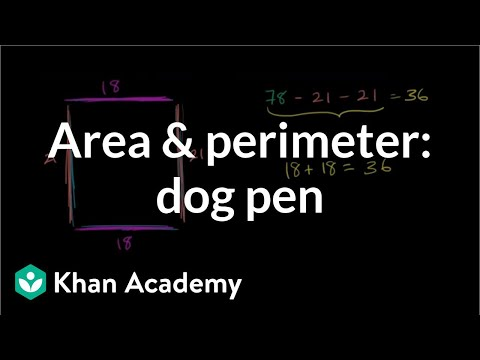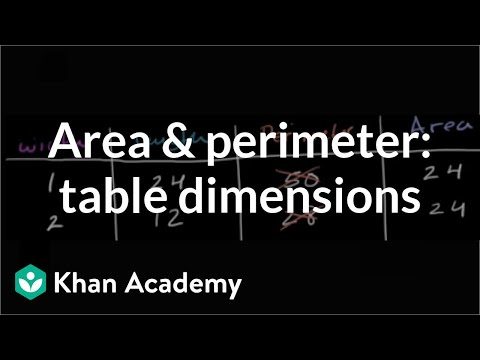# Finding perimeter when a side length is missing (Full video)

Description: Lindsay finds the perimeter of a figure when a side length is not given. Created by Lindsay Spears. Perimeter is the distance all the way around the outside of a shape. So to find that, what we can do is figure out how long is this side, and then combine it with the length of this side, and combine it with the length of this side, and once we combine all of the side lengths, we'll have the perimeter, or the distance around the outside of the figure. So we have five centimeters, plus, moving down the side here, we have another three centimeters, and then going across, next distance is four centimeters.

### Other videos you might be interested in### Area & perimeter word problem: dog pen (Full video)